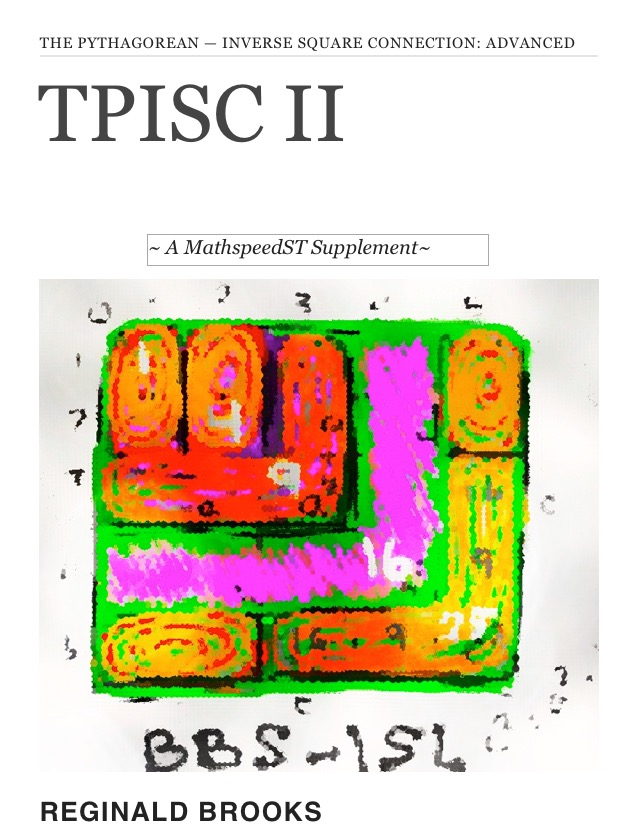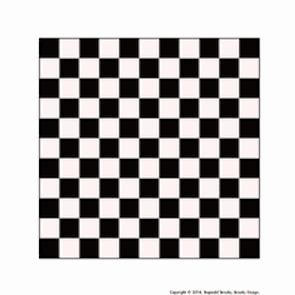# TPISC II: Advanced: The Pythagorean — Inverse Square Connection ~A MathspeedST Supplement~Statement

Introducing TPISC II: Advanced, an ebook. TPISC is a supplement to MathspeedST (published in November, 2013).

While LightspeedST addressed the ultimate question: “Why is the speed of light constant?”MathspeedST addressed the fundamental, underlying question: “How can information be ubiquitous, effectively traveling FASTER than the speed of light?”

TPISC describes the Pythagorean Triangles (Triples) and their ubiquitous connection to the unfolding Inverse Square Law (ISL) as depicted in the BBS-ISL Matrix.

The BBS-ISL Matrix (Brooks Base Square Matrix) presents the ISL as a natural, whole integer number matrix grid that expands to infnity.

TPISC is also, by definition, grounded in natural, whole integer numbers forming the sides of the Pythagorean Triples (PT). The PTs also expand to infinity and are deeply embedded within the BBS-ISL Matrix throughout their co-expansion. Enter TPISC.

TPISC I: Basics provided the simple, introductory geometrical (Visual) and algebraic (Calculation) mathematical basis for the accounting and distribution of all Pythagorean Triple Triangles — primitive and non-primitive — directly on the BBS-ISL Matrix.

TPISC II: Advanced greatly expands upon the simple, introductory geometrical (Visual) and algebraic (Calculation) mathematical basis presented in TPISC I: Basics. By further development of the Dickson Method (DM), the Expanded Dickson Method (EDM) is fully presented. Parsing the r-sets, and the s-sets and t-sets that result, onto the BBS-ISL Matrix reveals profound connections between the basic layout of the matrix and the distribution of the Pythagorean Triples. A complete geometric visualization is realized and this leads ultimately to the r-set Flow Chart that simplifies the entire distribution of ALL Pythagorean Triples — primitive and non-primitive PTs.

This interactive ebook brings together under one publication the intersection of two geometries: The Pythagorean Triangles and the BBS-ISL Matrix under the title TPISC II: Advanced. It includes hundreds of images, a number of videos and lots of test-yourself, interactive widgets in presenting the layout, proofs, and patterns of the Pythagorean Triples and their ISL-derivation and, ultimately, the mathematical basis of their connection with the matrix. Ages 13 and up, or younger if you are up to the task. Basically, if you can add and subtract, meaning you can multiply and divide, you can easily gain access to TPISC. Both TPISC and the BBS-ISL Matrix are composed solely of natural, whole integer numbers. No decimals, fractions, integrals, differentials,.... Nothing you can't handle! But plenty in the Advanced form to keep you going for...(ever!). TPISC I: Basics (free) is definitely the place for most to start.

A new paradigm in the visualization of basic mathematics is presented!.

Description:

The Pythagorean - Inverse Square Law Connection — or how the Pythagorean Theorem is intimately embedded within the The Architecture Of SpaceTime (TAOST) as described by the BBS-ISL Matrix — is first and foremost the topic of this book.

This work is being presented precisely because the very nature of that geometric relationship of the Pythagorean Triangle (PT) is so intimately related to the Inverse Square Law (ISL), as depicted on the BBS-ISL Matrix, that a cause and effect relationship between the two is unavoidable

That the BBS-ISL Matrix also provides the simplest, most intuitively obvious proof to the Theorem directly on the grid only supports the argument. Every possible PT, and its proof, is visually and mathematical present. The Dickson Method confirms this.

TPISC I: Basics
TPISC I: The Pythagorean - Inverse Square Connection: Basics — a separate ebook — revealed the presence, proofs and and overall distribution of the Pythagorean Triples (PTs) upon the BBS-ISL Matrix.  The distribution was shown as occurring on, and only on, each and every Row/Column that contained AREA Squares. Scan the Rows and if they contained Pair-Sets of these AREA Squares you would always find a PT there.

TPISC II: The Pythagorean - Inverse Square Connection: Advanced profoundly enlarges upon the Dickson Method — giving the Extended Dickson Method — which has opened the doors to the real connection between the ISL and the PTs. Patterns of Fractal-like Relationships, is a descriptive phrase loaded with the implications that the PTs, while ubiquitously embedded throughout the infinite BBS-ISL Matrix, grow within it in a profoundly fractal-like pattern. Parsing these into r-set and s-set groups becomes key in determining the pattern distribution of ALL PTs.

Every grid cell # within the BBS-ISL Matrix is determined by the Prime Diagonal #s, as is every possible PT.

The PTs, just like the circle and square, define the ISL!

So in the end, what are we to make of all this?
Why the BBS-ISL Matrix — which begs the question: Why the Inverse Square Law (ISL) — and why the  Pythagorean Triples?

And so what if they are both made up of whole integer #s?
And so what if they both expand to infinity?
And so what if the the PTs seem to be all inter-connected?
And so what if the Pts seem to be infinitely embedded within the BBS-ISL Matrix
And so what if there is a pattern to how they are embedded?
And so what if they both share a common pattern of 90° right-angle isosceles triangle relationships?
And so what if the ISL informs all of SpaceTime?
And so what if the ISL thus informs all expressions of energy as matter & waves — particles & fields?
And so what if all such interactions — including those of growth — is predicated on the unfolding of the ISL-dependent SpaceTime?
And so what if those simple PTs — pattern- peppered throughout the BBS-ISL Matrix — are laying down networking nodes of optimal 90° right-angle connections amongst the more widely distributed ISL background structure?

Are not the PTs there to organize it all?

TPISC, The Pythagorean — Inverse Square Connection , is about shining some light on how Nature ubiquitously builds combining simple, elemental forms into sentient constructs able to reflect the light all the way back home!

1. Introduction 2. The Underlying Question 3. TAOST (The Architecture Of SpaceTime) 4. TCAOP(The Complete Absence Of Primes) 5. Interconnectedness 6. Conclusion 7. Links & References 8. Appendix 9. Dyamic Interactive BBS-ISL matrix 10. Numbers of Inevitability 11. AFPOP: A Fresh Piece Of Pi(e)...and the Square Root of 2, too. 12. Next: BBS - ADVANCED (preview) 13. Review

Platforms: This is a multi-touch, interactive ebook made with iBooks Author and published in the Apple iBookstore. Currently, it requires an iPad, or OS Mavericks running on a Mac, and the free iBooks app to download and read.

Multi-touch, interactive eBook: TPISC II: Advanced ~ eBook

Selected SCREENSHOTS for TPISC II: Advanced

(NOTE: iBooks are available for reading on your Mac or iOS device.)

Artist Link in iTunes iBookstore: Reginald Brooks~---

~ MathspeedST: The Ubiquitous Information of Numbers white paper
~ LightspeedST: Leapfrogging @ The Speed of Light
T H E   U B I Q U I T O U S   I N F O R M A T I O N    O F    N U M B E R S new media net.art project
~ MathspeedST: Leapfrogging Lightspeed FASTER than The Speed of Light MOVIES
The contents of MathspeedST and LightspeedST, as well as most of the TPISC Series, can be viewed as White Papers in the Art Theory 101 links below.

Relevant White Papers by the Author

http://www.brooksdesign-ps.net/Reginald_Brooks/Code/Html/arthry5.htm

titles and topics:

### LightspeedST: The Complete New Media net.art project (HOME)

KEYWORDS TAGS:
TPISC, The Pythagorean - Inverse Square Connections, Pythagorean Triangles, Pythagorean Triples, primitive Pythagorean Triples, non-primitive Pythagorean Triples, Pythagorean Theorem, Pythagorus Theorem, The Dickson Method, BBS-ISL Matrix, Expanded Dickson Method, r-sets, s-set, t-sets, Pair-sets, geometric proofs, MathspeedST, leapfrogging LightspeedST FASTER than the speed of light, Brooks (Base) Square- Inverse Square Law (ISL), BBS-ISL matrix grid, The Architecture Of SpaceTime (TAOST), The Conspicuous Absence Of Primes (TCAOP), A Fresh Piece Of Pi(e), AFPOP, Numbers of Inevitability,LightspeedST, Teachers, Educators and Students (TES), number theory, ubiquitous information, FASTER than the speed of light, primes, prime numbers, fractals, mathematics, Universe, cosmos, patterns in number.

Art Theory 101 / White Papers Index
 PIN: Pattern in Number...from primes to DNA. | PIN: Butterfly Primes...let the beauty seep in. | PIN: Butterfly Prime Directive...metamorphosis. | PIN: Butterfly Prime Determinant Number Array (DNA) ~conspicuous abstinence~. | GoDNA: the Geometry of DNA (axial view) revealed. | SCoDNA: the Structure and Chemistry of DNA (axial view). | The LUFE Matrix | The LUFE Matrix Supplement | The LUFE Matrix: Infinite Dimensions | The LUFE Matrix: E=mc2 | Dark Matter=Dark Energy | The History of the Universe in Scalar Graphics | The History of the Universe_update: The Big Void | Quantum Gravity ...by the book | The Conservation of SpaceTime | LUFE: The Layman's Unified Field Expose` | GoMAS: The Geometry of Music, Art and Structure ...linking science, art and esthetics. Part I | Brooks (Base) Square (BBS): The Architecture of Space-Time (TAOST) and The Conspicuous Absence of Primes (TCAOP) - a brief introduction to the series net.art index | netart01: RealSurReal...aClone, 2001 | netart02: Hey!Ufunk'n with my DNA? | netart03: 9-11_remembered | netart04: Naughty Physics (a.k.a. The LUFE Matrix) | netart05: Your sFace or Mine? | netart06: Butterfly Primes | netart07: Music-Color-ISL | netart08: BBS-ISL matrix | netart09: BBS-interactive (I) | netart10: Sunspots and Solar Flares | netart11: Music-Color-ISL (II-III) | Art Theory 101: PIN, DNA, LUFE Matrix, GoMAS, BBS index | home Copyright 2015-19, Reginald Brooks, Brooks Design. All rights reserved. iTunes, iTunes Store, iBooks, iBooks Store, iBooks Author, Mac OS are registered® trademarks of Apple Inc. and their use on this webpage does not reflect endorsement by Apple Inc.